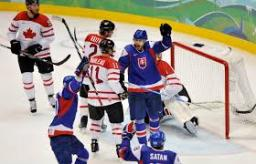# Tournament 3524

Four hockey teams scored 337 goals in the tournament. The second team scored 16 goals less than the first, the third 17 less than the second, and the fourth 30 goals less than the second. How many goals did each team score?

a =  108
b =  92
c =  75
d =  62

### Step-by-step explanation:

a+b+c+d=337
b = a-16
c = b-17
d = b-30

a+b+c+d = 337
a-b = 16
b-c = 17
b-d = 30

Row 2 - Row 1 → Row 2
a+b+c+d = 337
-2b-c-d = -321
b-c = 17
b-d = 30

Row 3 - 1/-2 · Row 2 → Row 3
a+b+c+d = 337
-2b-c-d = -321
-1.5c-0.5d = -143.5
b-d = 30

Row 4 - 1/-2 · Row 2 → Row 4
a+b+c+d = 337
-2b-c-d = -321
-1.5c-0.5d = -143.5
-0.5c-1.5d = -130.5

Row 4 - -0.5/-1.5 · Row 3 → Row 4
a+b+c+d = 337
-2b-c-d = -321
-1.5c-0.5d = -143.5
-1.3333d = -82.6667

d = -82.66666667/-1.33333333 = 62
c = -143.5+0.5d/-1.5 = -143.5+0.5 · 62/-1.5 = 75
b = -321+c+d/-2 = -321+75+62/-2 = 92
a = 337-b-c-d = 337-92-75-62 = 108

a = 108
b = 92
c = 75
d = 62

Our linear equations calculator calculates it.Did you find an error or inaccuracy? Feel free to write us. Thank you!

Tips for related online calculators
Do you have a system of equations and looking for calculator system of linear equations?